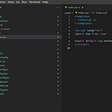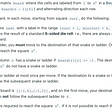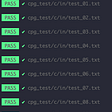# Opening

Listed as easy, description:

Given an array `nums`. We define a running sum of an array as `runningSum[i] = sum(nums…nums[i])`.

Return the running sum of `nums`.

Example 1:

`Input: nums = [1,2,3,4]Output: [1,3,6,10]Explanation: Running sum is obtained as follows: [1, 1+2, 1+2+3, 1+2+3+4].`

Example 2:

`Input: nums = [1,1,1,1,1]Output: [1,2,3,4,5]Explanation: Running sum is obtained as follows: [1, 1+1, 1+1+1, 1+1+1+1, 1+1+1+1+1].`

Example 3:

`Input: nums = [3,1,2,10,1]Output: [3,4,6,16,17]`

Constraints:

• `1 <= nums.length <= 1000`
• `-10^6 <= nums[i] <= 10^6`

# Solution

If this problem is challenging, it’s the fact that you have to keep track of a few invariants.

`class Solution:    def runningSum(self, nums: List[int]) -> List[int]:        ans = []        sum = 0        for i in range(len(nums)):            for j in range(i+1):                sum += nums[j]            ans.append(sum)            sum = 0                    return ans`

I just gave you the answer. Well, it was the first thing I came up with. Since I’m trying to do as many as possible, I leave the optimization to you. Or maybe I’ll come back to it another time.

You create an empty list to store your answer in and set the sum variatble to zero. The ans variable is what you’ll return.

The part you want to pay attention to is the double loop. The first loop will dictate how far the second loop goes. If there are 5 numbers, then the second loop will iterate until it reaches to 5 numbers.

The +1 in the second loop is important because it tells the program to consider the last element for summation. The sum variable gets reset to 0 after each i loop.

Of course, you’ll want to add the summed variable to the ans list before you reset it. It’s slow but it works.

--

--

--

I write just in case death knocks

Love podcasts or audiobooks? Learn on the go with our new app.

## Starting with Nuxt.js## On starting a Node.js Guild## React Native Do’s And Don’t## BookMyShow Clone In 4 Days## Adding Dark Mode to My React App## Why and how to structure Features in Modules in Angular## Desktop Team Proof of Work, November 2021## Cassy

I write just in case death knocks

## Is pair programming a match for you?